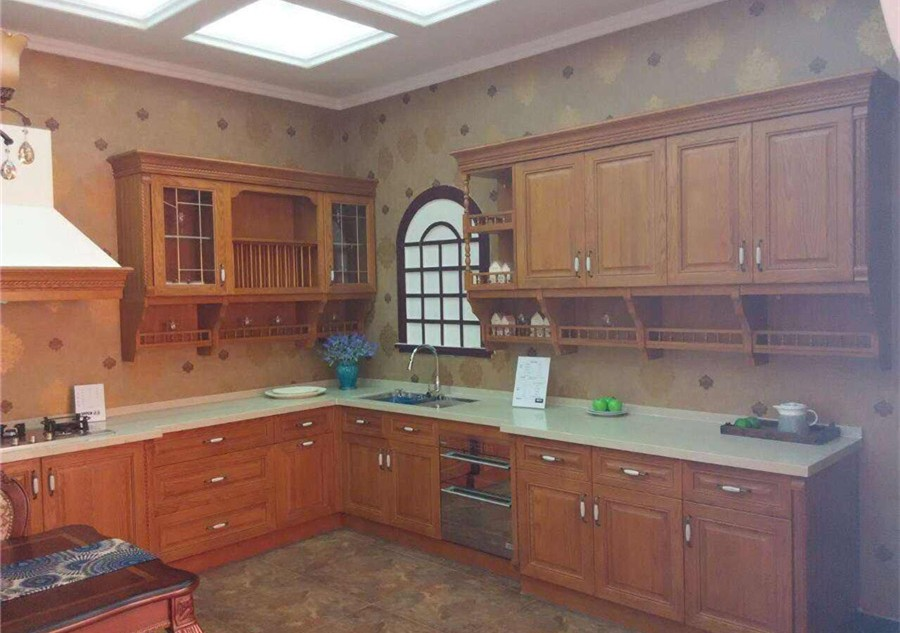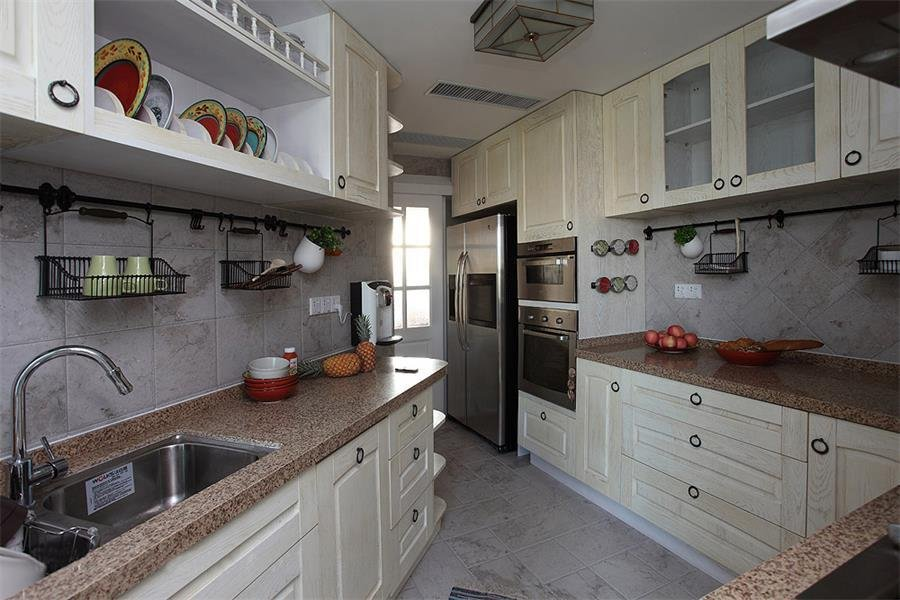|

# 新思路装饰:买橱柜时一定要问的十二个问题

第一招，要问柜体板的厚度。目前市场上有16毫米、18毫米等厚度规格的板材，厚度不同成本相差很大，仅此一项18厚的比16厚板材成本高出百分之7。18毫米厚板材做出的橱柜 使用寿命能延长一倍以上，保证门板不变形，保护台面不开裂。消费者看样品时一定要认真了解材料的构成情况，做到心中有数。

第二招，要问是独立柜体还是整组的柜体连在一起。整组连装的橱柜会影响牢度，因此消费者在选购整体橱柜时要问清楚，二者使用寿命和稳定性相差2-3倍，成本相差百分之5。消费者可以通过包装和装好的柜体来鉴别，如果独立柜体单柜组装的话，每个柜子都应该有一个独立的包装;消费者还可以在柜体安装好台面未安装时观察出来。

第三招，要问柜体板的颜色。市面上的柜体颜色五花八门，最主要的有暖白、灰白、冷白等，颜色不同成本不同，其中暖白板成本最高，高于其他饰面色百分之4。暖白饰面色泽柔和、饱满、美观大方是国际流行的箱体色彩，更是在环保、耐污、防霉、抗变色等诸多物理性能上大大超越了单调、发白的国产灰白、冷白饰面，用起来更干净、更安全。当然由于价格原因，也有的消费者会选择灰白或冷白柜体。第四招，要问后背板是单面封还是双面封。为节约成本，有的厂家偷工减料，对后背板只做单面封，看不到的一面是裸露的。单面封后背板容易潮湿、发霉，也容易释放甲醛，造成污染，因此一定要双面封的。

第五招，要问是否有防蟑静音封边。使用防蟑静音封边的柜体，柜门关闭时冲击力得以缓解，消除噪音，防止蟑螂等虫进入，有没有防蟑封边成本相差百分之3。还要查看台面板、门板、箱体和密封条、防撞条是否经机器模压处理，是否正反两面一次压制而成。密封条封闭不严会造成油烟、灰尘、昆虫进入。

第六招，要问封边胶水品牌。进口胶水不含或很少含有甲醛，因此不会产生加工污染，在耐用性上也可以保证更长时间不脱胶，防止甲醛泄漏。知名厂家会采用牛头、胶王、国民淀粉等进口品牌封边胶水。第七招，要问清人造石的成分。适合做厨房的台面的材料，有防火板、人造石、天然大理石、花岗岩、不锈钢等，其中以人造石台面的性能价格比最好。便宜的台面碳酸钙的成分高，容易开裂。目前市场上用的较多的是复合亚克力、纯亚克力等。复合亚克力中亚克力成分一般在百分之20左右为最佳比例。

第八招，要问清人造石是否做到无尘安装。过去不少厂家会在安装现场进行人造石打磨，造成居室环境污染，是消费者始料不及的。现在一些领先的橱柜厂商已经意识到了这一点，比如我乐春节后已经实现了人造石现场无粉尘施工的服务升级。如果你选择的橱柜厂家是有尘打磨的话，你一定要选择在地板、油漆进场前安装台面，否则就得再花钱做二次保洁了。

第九招，要问敢不敢提供材料原产地保证。一套橱柜由门板、柜体、人造石、五金配件等一系列部件构成，进口材料与国产材料存在很大的价差，同是国产材料，有品牌的和无品牌的价格相差也很大。因此要问清原产地，并在合同上按其宣传口径写上原产地保证。如查证不符，消费者有权索要双倍货款。第十招，要问成品能不能提供检测报告。橱柜也是家具产品，国家有明文规定要出具成品检测报告并明示甲醛含量。有的厂家只能提供原材料检验报告，但原材料环保不等于成品环保，只有成品合格才能证明其产品合格。因此消费者在购买时可以向商家索要，也可以把商家出示的质检报告编号记录下来打电话到质检部门核查真伪。

第十一招，要问保修期多长。不要只关心产品的价格和款式，能否能提供优质的售后服务是厂家实力的表现。比如保修年限，有的厂家是一年，有的厂家是二年，也有的是五年。敢于保五年的厂家，在用材、制造等环节一定会要求更高，对于消费者来讲也最实惠。许多小厂价格很便宜，但保修很短或者就没有保修，一但出了问题很难得到合理解决，所以消费者在订购橱柜时一定要问清楚产品保修等问题。正规厂家应该有书面的保修说明书，消费者应在仔细阅读后做决定。

第十二招，要问能不能参观工厂。漂亮展厅不代表雄厚实力。橱柜是一个完整的工业化产业，需要具备工业基础。有些公司宣传资料上的厂房照片、机器设备都是虚拟的，或是拿来的，其实很可能是个手工作坊式的小厂。机械设备对产能和工件质量有很大的影响，国外大品牌企业，几乎全部装备重型机械生产线。哪怕你不是真的想去也抛出这个问题，看对方是否欢迎你去的态度来做判断。

综上所述，在购买橱柜时，一定要认真地考虑各环节的因素。消费者在选择橱柜时，应尽量选择较佳的厂商或者商家。

`声明：本文由入驻焦点开放平台的作者撰写，除焦点官方账号外，观点仅代表作者本人，不代表焦点立场错误信息举报电话： 400-099-0099，邮箱：jubao@vip.sohu.com，或点此进行意见反馈，或点此进行举报投诉。`A B C D E F G H J K L M N P Q R S T W X Y Z
A - B - C - D - E
• A
• 鞍山
• 安庆
• 安阳
• 安顺
• 安康
• 澳门
• B
• 北京
• 保定
• 包头
• 巴彦淖尔
• 本溪
• 蚌埠
• 亳州
• 滨州
• 北海
• 百色
• 巴中
• 毕节
• 保山
• 宝鸡
• 白银
• 巴州
• C
• 承德
• 沧州
• 长治
• 赤峰
• 朝阳
• 长春
• 常州
• 滁州
• 池州
• 长沙
• 常德
• 郴州
• 潮州
• 崇左
• 重庆
• 成都
• 楚雄
• 昌都
• 慈溪
• 常熟
• D
• 大同
• 大连
• 丹东
• 大庆
• 东营
• 德州
• 东莞
• 德阳
• 达州
• 大理
• 德宏
• 定西
• 儋州
• 东平
• E
• 鄂尔多斯
• 鄂州
• 恩施
F - G - H - I - J
• F
• 抚顺
• 阜新
• 阜阳
• 福州
• 抚州
• 佛山
• 防城港
• G
• 赣州
• 广州
• 桂林
• 贵港
• 广元
• 广安
• 贵阳
• 固原
• H
• 邯郸
• 衡水
• 呼和浩特
• 呼伦贝尔
• 葫芦岛
• 哈尔滨
• 黑河
• 淮安
• 杭州
• 湖州
• 合肥
• 淮南
• 淮北
• 黄山
• 菏泽
• 鹤壁
• 黄石
• 黄冈
• 衡阳
• 怀化
• 惠州
• 河源
• 贺州
• 河池
• 海口
• 红河
• 汉中
• 海东
• 怀来
• I
• J
• 晋中
• 锦州
• 吉林
• 鸡西
• 佳木斯
• 嘉兴
• 金华
• 景德镇
• 九江
• 吉安
• 济南
• 济宁
• 焦作
• 荆门
• 荆州
• 江门
• 揭阳
• 金昌
• 酒泉
• 嘉峪关
K - L - M - N - P
• K
• 开封
• 昆明
• 昆山
• L
• 廊坊
• 临汾
• 辽阳
• 连云港
• 丽水
• 六安
• 龙岩
• 莱芜
• 临沂
• 聊城
• 洛阳
• 漯河
• 娄底
• 柳州
• 来宾
• 泸州
• 乐山
• 六盘水
• 丽江
• 临沧
• 拉萨
• 林芝
• 兰州
• 陇南
• M
• 牡丹江
• 马鞍山
• 茂名
• 梅州
• 绵阳
• 眉山
• N
• 南京
• 南通
• 宁波
• 南平
• 宁德
• 南昌
• 南阳
• 南宁
• 内江
• 南充
• P
• 盘锦
• 莆田
• 平顶山
• 濮阳
• 攀枝花
• 普洱
• 平凉
Q - R - S - T - W
• Q
• 秦皇岛
• 齐齐哈尔
• 衢州
• 泉州
• 青岛
• 清远
• 钦州
• 黔南
• 曲靖
• 庆阳
• R
• 日照
• 日喀则
• S
• 石家庄
• 沈阳
• 双鸭山
• 绥化
• 上海
• 苏州
• 宿迁
• 绍兴
• 宿州
• 三明
• 上饶
• 三门峡
• 商丘
• 十堰
• 随州
• 邵阳
• 韶关
• 深圳
• 汕头
• 汕尾
• 三亚
• 三沙
• 遂宁
• 山南
• 商洛
• 石嘴山
• T
• 天津
• 唐山
• 太原
• 通辽
• 铁岭
• 泰州
• 台州
• 铜陵
• 泰安
• 铜仁
• 铜川
• 天水
• 天门
• W
• 乌海
• 乌兰察布
• 无锡
• 温州
• 芜湖
• 潍坊
• 威海
• 武汉
• 梧州
• 渭南
• 武威
• 吴忠
• 乌鲁木齐
X - Y - Z
• X
• 邢台
• 徐州
• 宣城
• 厦门
• 新乡
• 许昌
• 信阳
• 襄阳
• 孝感
• 咸宁
• 湘潭
• 湘西
• 西双版纳
• 西安
• 咸阳
• 西宁
• 仙桃
• 西昌
• Y
• 运城
• 营口
• 盐城
• 扬州
• 鹰潭
• 宜春
• 烟台
• 宜昌
• 岳阳
• 益阳
• 永州
• 阳江
• 云浮
• 玉林
• 宜宾
• 雅安
• 玉溪
• 延安
• 榆林
• 银川
• Z
• 张家口
• 镇江
• 舟山
• 漳州
• 淄博
• 枣庄
• 郑州
• 周口
• 驻马店
• 株洲
• 张家界
• 珠海
• 湛江
• 肇庆
• 中山
• 自贡
• 资阳
• 遵义
• 昭通
• 张掖
• 中卫

1室1厅1厨1卫1阳台

1
2
3
4
5

0
1
2

1

1

0
1
2
3报名成功，资料已提交审核A B C D E F G H J K L M N P Q R S T W X Y Z
A - B - C - D - E
• A
• 鞍山
• 安庆
• 安阳
• 安顺
• 安康
• 澳门
• B
• 北京
• 保定
• 包头
• 巴彦淖尔
• 本溪
• 蚌埠
• 亳州
• 滨州
• 北海
• 百色
• 巴中
• 毕节
• 保山
• 宝鸡
• 白银
• 巴州
• C
• 承德
• 沧州
• 长治
• 赤峰
• 朝阳
• 长春
• 常州
• 滁州
• 池州
• 长沙
• 常德
• 郴州
• 潮州
• 崇左
• 重庆
• 成都
• 楚雄
• 昌都
• 慈溪
• 常熟
• D
• 大同
• 大连
• 丹东
• 大庆
• 东营
• 德州
• 东莞
• 德阳
• 达州
• 大理
• 德宏
• 定西
• 儋州
• 东平
• E
• 鄂尔多斯
• 鄂州
• 恩施
F - G - H - I - J
• F
• 抚顺
• 阜新
• 阜阳
• 福州
• 抚州
• 佛山
• 防城港
• G
• 赣州
• 广州
• 桂林
• 贵港
• 广元
• 广安
• 贵阳
• 固原
• H
• 邯郸
• 衡水
• 呼和浩特
• 呼伦贝尔
• 葫芦岛
• 哈尔滨
• 黑河
• 淮安
• 杭州
• 湖州
• 合肥
• 淮南
• 淮北
• 黄山
• 菏泽
• 鹤壁
• 黄石
• 黄冈
• 衡阳
• 怀化
• 惠州
• 河源
• 贺州
• 河池
• 海口
• 红河
• 汉中
• 海东
• 怀来
• I
• J
• 晋中
• 锦州
• 吉林
• 鸡西
• 佳木斯
• 嘉兴
• 金华
• 景德镇
• 九江
• 吉安
• 济南
• 济宁
• 焦作
• 荆门
• 荆州
• 江门
• 揭阳
• 金昌
• 酒泉
• 嘉峪关
K - L - M - N - P
• K
• 开封
• 昆明
• 昆山
• L
• 廊坊
• 临汾
• 辽阳
• 连云港
• 丽水
• 六安
• 龙岩
• 莱芜
• 临沂
• 聊城
• 洛阳
• 漯河
• 娄底
• 柳州
• 来宾
• 泸州
• 乐山
• 六盘水
• 丽江
• 临沧
• 拉萨
• 林芝
• 兰州
• 陇南
• M
• 牡丹江
• 马鞍山
• 茂名
• 梅州
• 绵阳
• 眉山
• N
• 南京
• 南通
• 宁波
• 南平
• 宁德
• 南昌
• 南阳
• 南宁
• 内江
• 南充
• P
• 盘锦
• 莆田
• 平顶山
• 濮阳
• 攀枝花
• 普洱
• 平凉
Q - R - S - T - W
• Q
• 秦皇岛
• 齐齐哈尔
• 衢州
• 泉州
• 青岛
• 清远
• 钦州
• 黔南
• 曲靖
• 庆阳
• R
• 日照
• 日喀则
• S
• 石家庄
• 沈阳
• 双鸭山
• 绥化
• 上海
• 苏州
• 宿迁
• 绍兴
• 宿州
• 三明
• 上饶
• 三门峡
• 商丘
• 十堰
• 随州
• 邵阳
• 韶关
• 深圳
• 汕头
• 汕尾
• 三亚
• 三沙
• 遂宁
• 山南
• 商洛
• 石嘴山
• T
• 天津
• 唐山
• 太原
• 通辽
• 铁岭
• 泰州
• 台州
• 铜陵
• 泰安
• 铜仁
• 铜川
• 天水
• 天门
• W
• 乌海
• 乌兰察布
• 无锡
• 温州
• 芜湖
• 潍坊
• 威海
• 武汉
• 梧州
• 渭南
• 武威
• 吴忠
• 乌鲁木齐
X - Y - Z
• X
• 邢台
• 徐州
• 宣城
• 厦门
• 新乡
• 许昌
• 信阳
• 襄阳
• 孝感
• 咸宁
• 湘潭
• 湘西
• 西双版纳
• 西安
• 咸阳
• 西宁
• 仙桃
• 西昌
• Y
• 运城
• 营口
• 盐城
• 扬州
• 鹰潭
• 宜春
• 烟台
• 宜昌
• 岳阳
• 益阳
• 永州
• 阳江
• 云浮
• 玉林
• 宜宾
• 雅安
• 玉溪
• 延安
• 榆林
• 银川
• Z
• 张家口
• 镇江
• 舟山
• 漳州
• 淄博
• 枣庄
• 郑州
• 周口
• 驻马店
• 株洲
• 张家界
• 珠海
• 湛江
• 肇庆
• 中山
• 自贡
• 资阳
• 遵义
• 昭通
• 张掖
• 中卫• 手机• 分享
• 设计
免费设计
• 计算器
装修计算器
• 入驻
合作入驻
• 联系
联系我们
• 置顶
返回顶部